Next: Conclusion Up: Numerical examples Previous: Example 5

## Example 6, an elliptic system of logistic type

In this section we consider an optimal control problem for a semilinear elliptic equation of logistic type which was studied in Leung, Stojanovic [21,28]. The problem is to determine a distributed control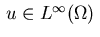that minimizes the functional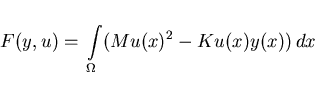(4.7)

subject to the elliptic state equation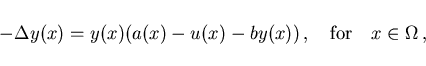(4.8)

homogeneous Neumann boundary conditions,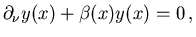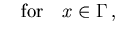(4.9)

and control and state inequality constraints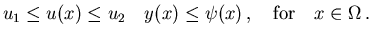(4.10)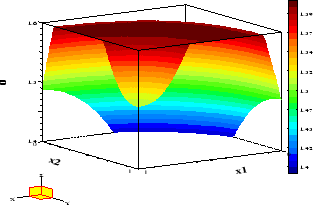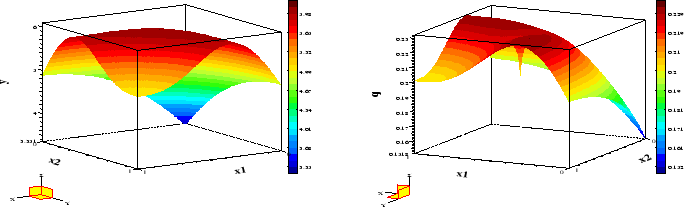Here,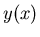denotes the population of a biological species,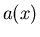a spatially dependent intrinsic growth rate,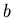the crowding effect, while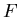denotes the difference between economic cost and revenue, with nonnegative constants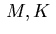. In [21,28] the function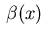was chosen as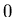. Numerical results for this case can be found in . Here, we consider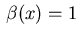if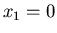or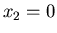and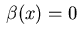otherwise. The goal is to find a control function which maximizes profit. A similar control problem with Dirichlet boundary conditions was recently studied by Cañada et al. .

The adjoint equations (2.10), (2.11) yield the following equations: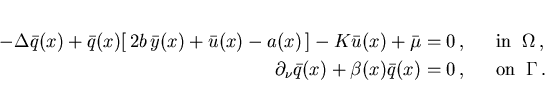For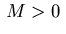, the minimum condition (2.18) gives the control law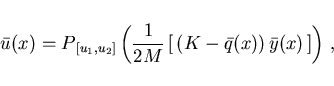(4.11)

where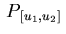denotes the projection operator on the interval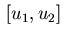.

The following concrete data were used: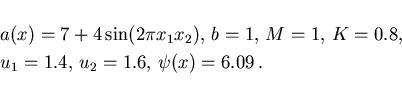Figure 11 displays the optimal control while Figure 12 shows the optimal state and adjoint variable. The reader may verify that the minimum condition given by the projection (4.11) holds with high accuracy. However, note that condition (2.7) imposed in  is not satisfied everywhere in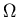.

Table 7: Information on solution of Example 6
 N+1 it CPU Acc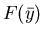50 29 104 8 -4.19322 100 32 2235 8 -4.27569 200 33 42543 8 -4.31709Next: Conclusion Up: Numerical examples Previous: Example 5
Hans D. Mittelmann
2000-10-06